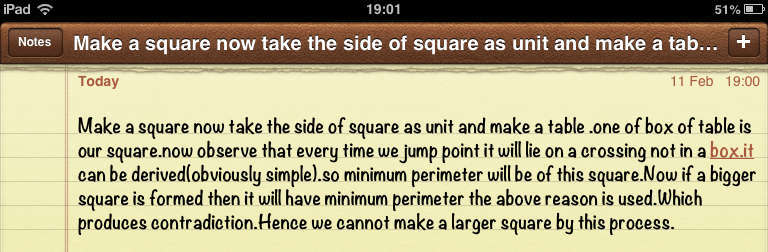Making a square larger

You are given four points (on a Euclidian plane) that make up the corners of a square.  You may change the positions of the points by a sequence of moves.  Each move changes the position of one point, say p, to a new location, say p’, by “skipping over” one of the other 3 points.  More precisely, p skips over a point q if it is moved to the diametrically opposed side of q.  In other words, a move from p to p’ is allowed if there exists a point q such that q = (p + p’) / 2.

Find a sequence of moves that results in a larger square.  Or, if no such sequence is possible, give a proof of why it isn’t possible.  (The new square need not be oriented the same way as the original square.  For example, the larger square may be turned 45 degrees from the original, and the larger square may have the points in a different order from in the original square.)